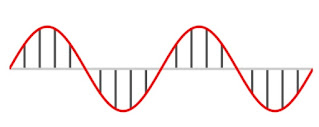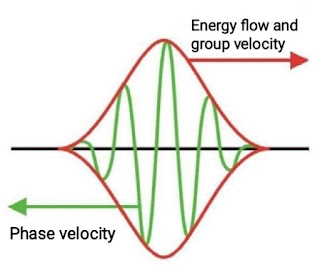# Phase Velocity | Group Velocity | Relation between Phase Velocity and Group Velocity for De Broglie Waves

Phase velocity, Group velocity, Relation, Difference , A wave travels through a medium, its velocity of advancement in the medium is called

## ▶Phase velocity

When a monochromatic wave travels through a medium, its velocity of advancement in the medium is called phase velocity. It is represented by vp and given by

v_p=\frac\omega k

Where, ω = angular velocity

k = 2π / λ (propagation constant)

Thus the Phase velocity is the velocity with which planes of constant phase advance through the medium.Phase velocity

## ▶Group velocity

When a group of waves travel in a diffuse medium, the phase velocities of its various components vary. Thus, the velocity with which the maximum amplitude of the group advances through the medium is called the group velocity. It is represented by vg given by

v_g=\frac{\triangle\omega}{\triangle k}Group velocity

## ▶Relation between phase velocity and group velocity

Let u and vg be the phase velocity and group velocity for a non-relativistic free particle of mass m. If (λ) is the de-broglie wavelength and (ν) is the frequency of the wave, phase velocity is given by

u = ν.λ............(1)

According to de broglie hypothesis

λ = h / mv.........(2)

Total energy of free particle,

E_k=\frac1 2mv^2

also,

E=h\nu

\nu=\frac E h=\frac1 2\frac{mv^2}h........(3)

Substituting (λ) and (f) from equation (2) and (3) into Eq. (1), we get

u=\frac1 2\frac{mv^2}h.\frac h{mv}

u=\frac1 2v_g

This is the relation between phase velocity and group velocity for a non-relativistic free particle. Thus for a non-relativistic free particle the group velocity (vg) is twice of the phase velocity (u).

## Difference Between Phase Velocity and Group Velocity

 S.No. Phase Velocity Group Velocity 1. In a normal medium, the phase velocity is greater than the group velocity. In an anomalous medium, the group velocity is greater than the phase velocity. 2. Phase velocity is defined for both, the single waves and superimposed waves. The group velocity is defined only to the superimposed waves. 3. The phase velocity is the velocity of the wave with higher frequency. The group velocity is the velocity of the wave with lower frequency. 4. The Phase velocity refers to the velocity of a monochromatic wave. Group velocity is the velocity with which the entire group of waves would travel.